# Static MembersS2C Home « Static Members

We have used the static keyword throughout the lessons so far, everytime we use the `main()` method. In this lesson we investigate what static means and how to apply it to our classes. When we use the `static` keyword preceding our identifiers we are telling the compiler that this is a class member. What do we mean by this? Well class members apply to the class itself and are independent of any instances of the class, in fact our static members know nothing about the instances within our class. We can also use the `static` keyword to initiate a block of code on class load.

The following diagram shows a static variable, static initializer block and a static method: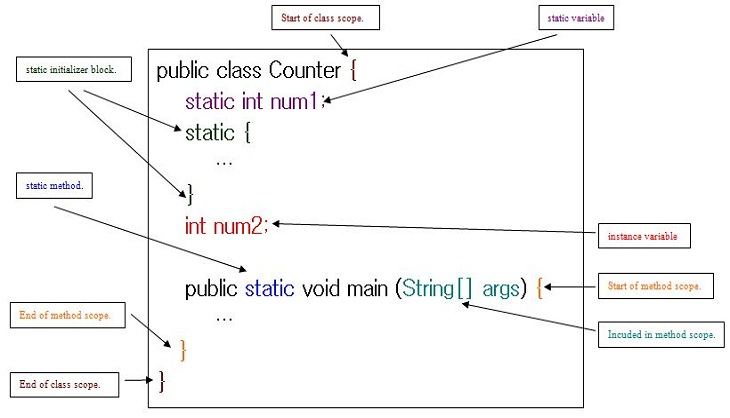We will start this lesson by looking at static variables, how we use them and their scope.

### Static VariablesTop

Java comes with three kinds of scope and we name variables according to the scope they reside in as detailed in the table below. In this section we focus on static variables and their scope.

staticStatic variables apply to the class as a whole and are declared within the class but outside a method.Exists for as long as the class it belongs to is loaded in the JVM.
instanceInstance variables apply to an instance of the class and are declared within the class but outside a method.Exists for as long as the instance of the class it belongs to.
localLocal variables apply to the method they appear in.Exists until the method it is declared in finishes executing.

Static variables are initialized as soon a class is loaded and before any instances of the class are instantiated.

Like instance variables, static variables are assigned a default value according to their type:

• object - `null`
• boolean - `false`
• char - `/u0000`
• integer types (`byte`, `short`, `int` and `long`) - `0`
• floating-point types (`float`, `double`) - `0.0`

Because static variables are independent of any instance they can be used by all instances of the class. To demonstrate what we mean by this lets write some code that keeps count of the instances we create.

``````
/*
A Donkey Class
*/
public class Donkey {
int count;

public Donkey() {
count++;  // Increment a counter
System.out.println("Our donkey count = " + count);
}
}

``````

Save and compile the file in directory   c:\_ObjectsAndClasses in the usual way. Lets write a new test class for our `Donkey`.

``````
/*
Test Class for Donkey
*/
public class DonkeyTest {

public static void main (String[] args) {
Donkey dan = new Donkey();
Donkey sam = new Donkey();
Donkey liam = new Donkey();
}
}

``````

Save, compile and run the file in directory   c:\_ObjectsAndClasses in the usual way.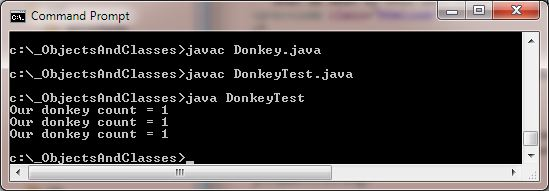The above screenshot shows the output of running our `Donkey` class. We created three `Donkey` instances and printed out a total after each. The total is always 1 as each time we create a new instance of `Donkey` we are creating a new `count` variable as this is an instance variable. Lets revamp our `Donkey` class and make the instance variable into a static variable:

``````
/*
A Donkey Class
*/
public class Donkey {
static int count;  // We made this static

public Donkey() {
count++;  // Increment a counter
// Prefix statics with the class name
System.out.println("Our donkey count = " + Donkey.count);
}
}

``````

Save and compile the file in directory   c:\_ObjectsAndClasses in the usual way and then rerun our `DonkeyTest` class.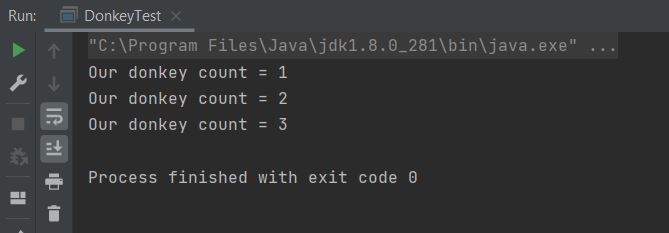The above screenshot shows the output of running our `Donkey` class. We created three `Donkey` instances and printed out a total after each. The total now gets gets incremented each time we create a new instance of `Donkey` just like we want. Static variables apply to the whole class and are initialized when the class is loaded. There is only one copy of each static variable in a class which is shared by all instances of that class. We prefix statics with the class name to avoid ambiguity and to highlight the fact that this is a static.

### Java ConstantsTop

We assign a java constant (a value that never changes) through the use of the `static` and `final` keywords. You must always assign a value to a static variable marked as `final` before you use it or the compiler complains. A static variable that is marked as `final` can never change once it has been assigned a value. So once assigned a value, trying to change a variable marked as `final` will cause a compiler error.

You can also use the `final` keyword to create final local variables, final instance variables, within parameter lists and for preventing inheritance/overriding.

So lets use some code to look at creating some Java constants.

``````
/*
Test class for Java Constants
*/
public class JavaConstants {
public static final double EULERS_CONSTANT = 2.718281828459045;  // Base of natural logarithms
public final static int SQUARE_OF_THREE;  // 3 * 3

public static void main (String[] args) {
System.out.println("Euler's Constant = " + EULERS_CONSTANT);
System.out.println("Three squared + " + SQUARE_OF_THREE);
}
}

``````

Save and compile the file in directory   c:\_ObjectsAndClasses in the usual way.The above screenshot shows the output of running the `JavaConstants` class. We got a compiler error as we forgot to initialize `SQUARE_OF_THREE`. Ok lets change the `JavaConstants` class.

``````
/*
Test class for Java Constants
*/
public class JavaConstants {
public static final double EULERS_CONSTANT = 2.718281828459045;  // Base of natural logarithms
public final static int SQUARE_OF_THREE = 9;  // 3 * 3

public static void main (String[] args) {
System.out.println("Euler's Constant = " + EULERS_CONSTANT);
SQUARE_OF_THREE = 9;    // Here we set the value of SQUARE_OF_THREE again
System.out.println("Three squared + " + SQUARE_OF_THREE);
}
}

``````

Save and compile the file in directory   c:\_ObjectsAndClasses in the usual way.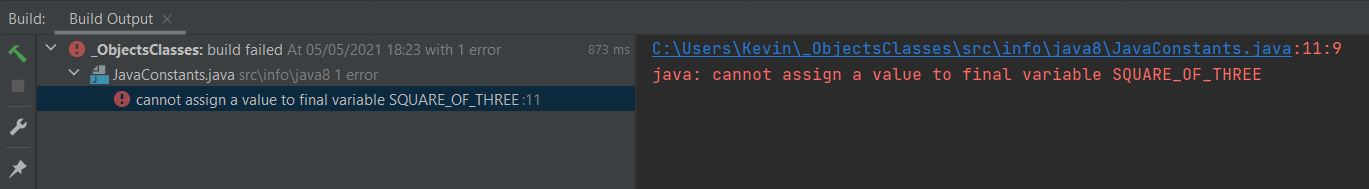The above screenshot shows the output of running the `JavaConstants` class. We got a compiler error as we initialized `SQUARE_OF_THREE`, and then tried to assign a value to if after this which we can't do. Lets remove the second assignment:

``````
/*
Test class for Java Constants
*/
public class JavaConstants {
public static final double EULERS_CONSTANT = 2.718281828459045;  // Base of natural logarithms
public final static int SQUARE_OF_THREE = 9;  // 3 * 3

public static void main (String[] args) {
System.out.println("Euler's Constant = " + EULERS_CONSTANT);
System.out.println("Three squared + " + SQUARE_OF_THREE);
}
}

``````

Save, compile and run the file in directory   c:\_ObjectsAndClasses in the usual way.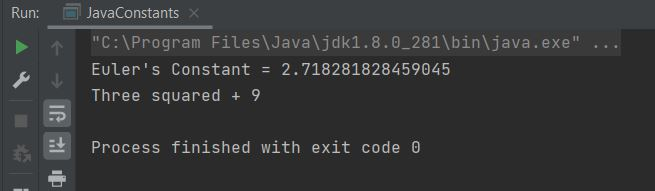The above screenshot shows the output of running the `JavaConstants` class.

#### Static Initializer BlocksTop

You can only initialize static variables in two ways: when you declare it or in a static initializer block. Static initializer blocks run as soon as a class is loaded, before static variables and static methods and are used to action things we want done before anything else. Lets see how static initializer blocks work with our Java constants:

``````
/*
Test class for Java Constants
*/
public class JavaConstants2 {
public static final double EULERS_CONSTANT;
public final static int SQUARE_OF_THREE;

/*
Static Initializer Block
*/
static {
EULERS_CONSTANT = 2.718281828459045;
SQUARE_OF_THREE = 9;
}

public static void main (String[] args) {
System.out.println("Euler's Constant = " + EULERS_CONSTANT);
System.out.println("Three squared + " + SQUARE_OF_THREE);
}
}

``````

Save, compile and run the file in directory   c:\_ObjectsAndClasses in the usual way.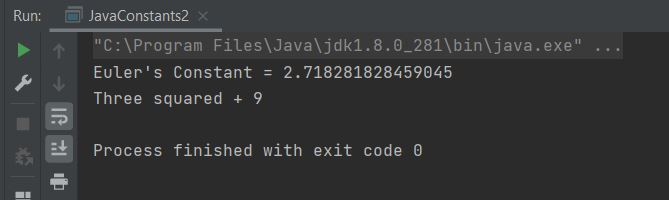The above screenshot shows the output of running the `JavaConstants2` class.

#### Java Constants ChecklistTop

• There is no way to designate a constant in Java; so the convention is to use all uppercase letters and separate words using the underscore ( `_` ) symbol when defining a constant.
• The `static` and `final` keywords can go either way round.
• You must initialize any static variable marked `final` before use, or the compiler throws an error. This can be done when declaring the static variable or in a static initailizer block.
• Once initialized any static variable marked `final` cannot have its value changed elsewhere or the compiler throws an error.

### Static MethodsTop

Static methods run without any instance of a class being involved, as static they belong to the class as a whole, and are accessed using the name of the class in the same way as static variables. Lets think about it, `the main()` method we have used since the start of these lessons to test our classes, runs before any instances of a class exist. In fact you cannot access the instance variables of a class from a static method within the class or the compiler gets upset. This makes sense as how would a static method know which instance variable to use, there is no reference. Its the same thing when you try to call a non-static method from a static method, the compiler doesn't like it. The static method has no way of knowing how to use any instance variables associated with the non-static method and has no way to reference which instance they belong to. Even if the method has no instance variables the compiler still won't allow it because in the future you may amend the method to include an instance variable. Lets look at these rules with some code:

``````
/*
Test class for static calls
*/
public class StatCalls {

public static void main (String[] args) {
int aSquare = squareNumber(5);   // Calling non-static method
System.out.println(aSquare);
}

/*
A method that squares and returns the passed integer
*/
int squareNumber(int number) {
int square = number * number;
return square;  // Here we use the return keyword to pass back a value
}
}

``````

Save, compile and run the file in directory   c:\_ObjectsAndClasses in the usual way.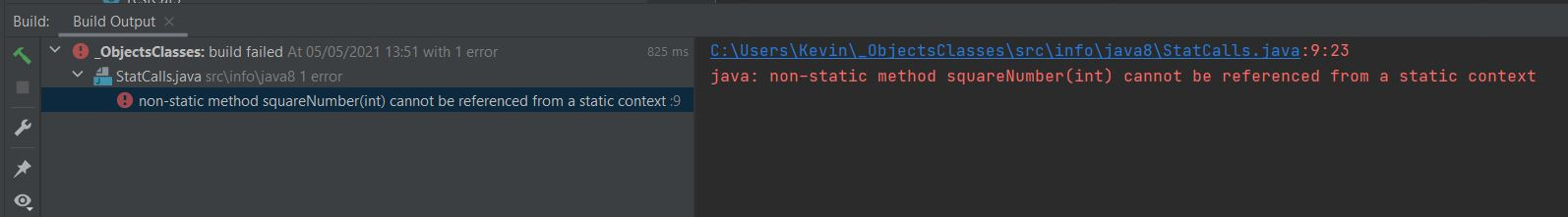The above screenshot shows the output of running our `StatCalls` class. The compiler doesn't like the fact that we are calling a non-static method from `main()` method. Lets look at using instance variables from a static method.

``````
/*
Test class for static calls
*/
public class StatCalls2 {
int number;

public static void main (String[] args) {
number = 5;  // Calling instance variable
int aSquare = StatCalls2.squareNumber(5);   // Calling static method
System.out.println(aSquare);
}

/*
A method that squares and returns the passed integer
*/
static int squareNumber(int number) {
int square = number * number;
return square;  // Here we use the return keyword to pass back a value
}
}

``````

Save, compile and run the file in directory   c:\_ObjectsAndClasses in the usual way.The above screenshot shows the output of running our `StatCalls2` class. The compiler doesn't like the fact that we are calling an instance variable from the `main()` method.

Of course there is no problem using instantiation from within your static method to access non-static content, or using a local reference from within your static method to access non-static content; otherwise you would never be able to get out of the `main()` method.

## Lesson 7 Complete

In this lesson we investigated what `static` means and how to apply it to our class members.

### What's Next?

In our final lesson of this section we go through enumerations which were introduced in java.

All the java lessons are listed below. Click a link to go to that lesson.